# Activation energy

Jump to: navigation, search

A concept originating in the theory of chemical reactions. It plays an important role in combustion theory.

The evolution of a chemical reaction is determined by its specific-reaction rate constant, usually denoted by. This quantity depends mainly on the temperature,. For a one-step chemical reaction, its functional dependence is given empirically by the Arrhenius expression:. Here,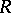is the universal gas constant,the activation energy andthe frequency factor for the reaction step;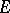is independent of, whilemay depend weakly (for example, polynomially) on.

At the molecular level, a chemical reaction is a collision process between reactant molecules, from which reaction products emerge. The molecules move on a potential-energy surface, whose shape is determined by a solution of the Schrödinger equation. A configuration of the reactant molecules corresponds to a local minimum in one region, and a configuration of the reaction products to a local minimum in another region, where the two minima are generally separated by a barrier in the potential-energy surface. At a saddle point on the barrier, the height of the surface above the energy of the reactant region assumes a minimum value. A collision of the reactant molecules can produce products only if the energy of the reactants (for example, their kinetic energy) exceeds this minimum height. The minimum barrier height defines the activation energy,. It is the energy the reactants must acquire before they can react. In practice, the activation energy is determined experimentally, by measuringat various values ofand making a best straight-line fit through the data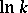versus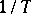.

## Activation-energy asymptotics.

Activation-energy asymptotics play an important role in combustion theory, in the study of diffusion flames.

A diffusion flame is a non-pre-mixed, quasi-steady, nearly isobaric flame, where most of the reaction occurs in a narrow zone. The structure of a diffusion flame, especially in a multi-step reaction, is very complicated, but can be analyzed in model problems by means of activation-energy asymptotics.

The small parameter in activation-energy asymptotics is the reciprocal of the Zel'dovich number. The Zel'dovich number,, is a non-dimensional measure of the temperature sensitivity of the reaction rate: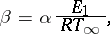wherewhile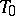and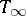denote the temperature far upstream and far downstream of the moving flame, respectively, andis a reference energy. The graph of the reaction rate has a single peak, which narrows and increases in magnitude and whose location approaches the fully burnt condition () as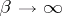.

The governing equations for the temperature and the concentration of the reaction-limiting component define a singular perturbation problem (cf. also Perturbation theory) as. The regular (or outer) expansion yields the temperature and concentration profiles in the convective-diffusive zone outside the flame, and the "singular" (or "inner" ) expansion the profiles in the reactive-diffusive zone inside the flame. The complete profiles are then found by the method of matched asymptotic expansions (cf. Perturbation theory).

Activation-energy asymptotics were first introduced in combustion theory by Yu.B. Zel'dovich and D.A. Frank-Kamenetskii [a1]. The method was formalized in [a2]. Many results are summarized in [a3].